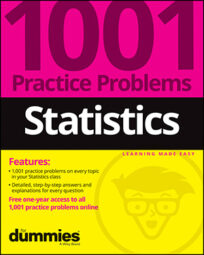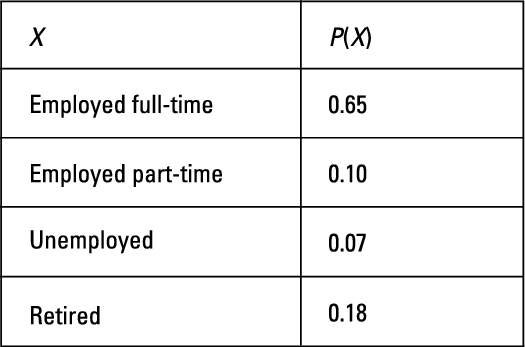##### Statistics: 1001 Practice Problems For Dummies (+ Free Online Practice)In the practice problems here, you will be finding probabilities for a random variable. The following table represents the probability distribution for X, the employment status of adults in a city.## Sample questions

1. If you select one adult at random from this community, what is the probability that the individual is employed part-time?

From the table, you see that 0.10 or 10% of the adults in the city are employed part-time. Using notation, this means that P(part-time) = 0.10.

2. If you select one adult at random from this community, what is the probability that the individual isn't retired?

Because total probability is always equal to 1, the probability that someone isn't retired is 1 minus the probability that the person is retired (which, according to the table, is 0.18 in this case). So the probability that the adult isn't retired is 1 – 0.18 = 0.82, or 82%. Using notation, this means that P(not retired) = 0.82.

3. If you select one adult at random from this community, what is the probability that the individual is working either part-time or full-time?